# AP Statistics : How to apply conditional probability

## Example Questions

### Example Question #1 : How To Apply Conditional Probability

How many different football teams of 11 members may be created from 13 men, without regard to the position played by any of the members?

120

13

65

78

11

78

Explanation:

There are 13 men to choose from in order to make an 11 member football team. Thus, the answer is set up as: 13 choose 11: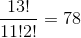.

### Example Question #14 : Independent And Dependent Events

 Number of EmailsProbability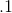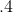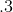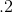The number of emails that Sam can respond to per hour is given by this probability distribution. What is the average number of emails that Sam respons to per hour?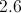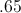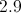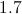Explanation:

The answer is found by multiplyingby the probability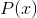: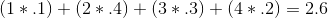emails

### Example Question #1 : How To Apply Conditional Probability

A child has a bag of marbles--red,blue, andyellow.  The child randomly selects one marble and gives it away. The child then selects a second marble.  What is the probability that both marbles selected were yellow?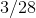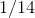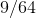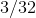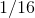Explanation:

The two events in this case are dependent (the result of the first event will affect the outcome probabilities of the second event). Find the probability of a particular outcome for both events by multiplying the probability of event A by the probability of event B given the result of A. In other words, multiply the probability of both events, remembering to account for the result of event A in determining the probability of event B.  Here, the probability of selecting a yellow marble the first time is 3/8, and the second time is 2/7.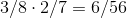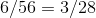### Example Question #1 : How To Apply Conditional Probability

There are 10 horses in a herd. 4 are males, 6 are females. 7 of the horses are brown while the remaining 3 are white. 1 male horse is white and 5 female horses are brown.

A horse is randomly selected from the herd. Given that the horse is brown, what is the probability that the horse is a male?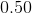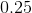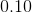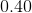Explanation:

This problem is a conditional probability because it asks for the probability of an event given that another event has occured.

The formula is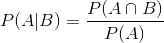It is important to correctly identify A and B and place them in the equation correctly.

If you read the equation out loud, it would be read "Probability of A given B equals the probability of A and B divided by the probability of B.

The problem asks for the the probability of a male given that the horse is brown. Therefore, A is male horse. B is brown horse.

Now, use the given information to fill in the equation.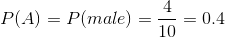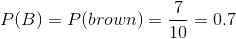If one male horse is white, there are 2 brown males out of the 10 horses total.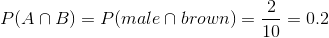Now solve the equation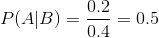### Example Question #1 : How To Apply Conditional Probability

There are 10 horses in a herd. 4 are males, 6 are females. 7 of the horses are brown while the remaining 3 are white. At least 1 male horse is white and 5 female horses are brown.

One horse is selected at random from the herd. What is the probability of selecting a female horse given that the horse selected is white?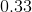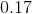Cannot be determined by the information given.Explanation:

This question asks for the probablity of an event given that one event has occured, meaning it is a conditional probability. The equation for a conditiona probabiliy is:It is important to properly assign the A and B variables according to the question. It helps to read the equation out loud: "The probability of A given B equals the probability of A and B divided by the proability of A."

We need to find the probability of a female given that the horse is white. Therefore, female horse is A, white horse is B.

So, find each probability needed for the equation.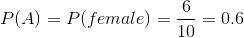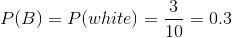If 6 of the 7 females are brown, then 1 horse out of 10 is a white female horse.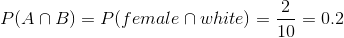Now, use the information to solve the equation.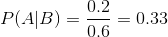### Example Question #1 : How To Apply Conditional Probability

There are 10 horses in a herd. 4 are males, 6 are females. 7 of the horses are brown while the remaining 3 are white. 1 male horse is white, 5 female horses are brown.

A horse is randomly selected from the herd. What is the probability that the horse chosen is brown or female?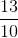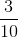Explanation:

This problem asks for the probability of one event or another, so the addition rule of probability is approriate. However, brown horse and female horse are not mutually exclusive events, because there are brown female horses. Therefore we must subtract the brown male horses to avoid double counting.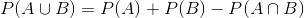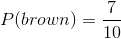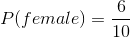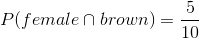Now, fill in the equation.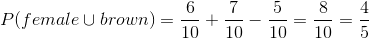### Example Question #19 : Independent And Dependent Events

The probability that there will be an accident on Highwayeach day is determined by the weather. If it's a dry day, there is a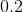% chance of an accident, and a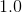% chance if it's a wet day. The radio says that there is a% chance of rain today. What is the probability that there will be an accident on Highwaytoday?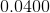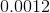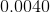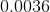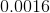Explanation: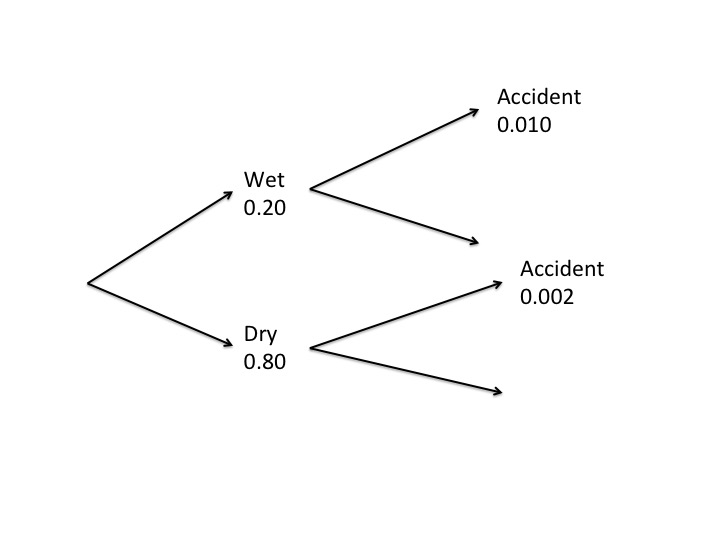The chance of an accident is dependent on the weather conditions, so that is the first branch on the tree diagram.

The probabilities at the next branch are conditional and the last two branches have the chance of accident.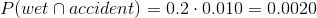and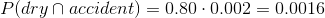,

so the total probability of an accident is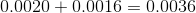.

### Example Question #1 : How To Apply Conditional Probability

There is a sample of 100 randomly selected children (60 of which are boys and 40 are girls).  They were asked if they liked to play football.  55 boys answered yes to the question and 5 of the girls aswered yes to the question.  What is the probability that a child said yes to the question give that they are a boy?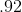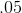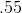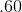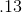The conditional probability formula is the probability of both events happening divided by the probability of the given event.  The probability of saying "yes" and also being a boy is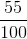.  The probability of being a boy in the sample is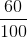.  So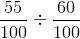=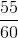=.